Check Full Chapter Explained - Continuity and Differentiability - Continuity and Differentiability Class 12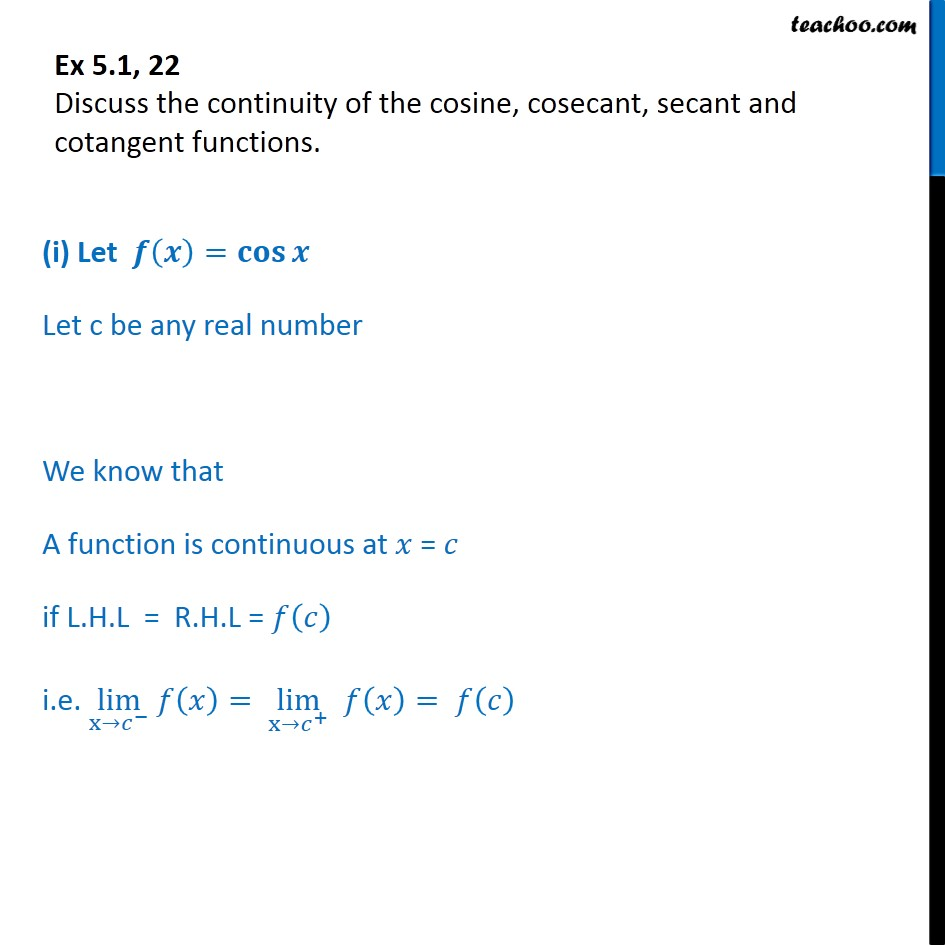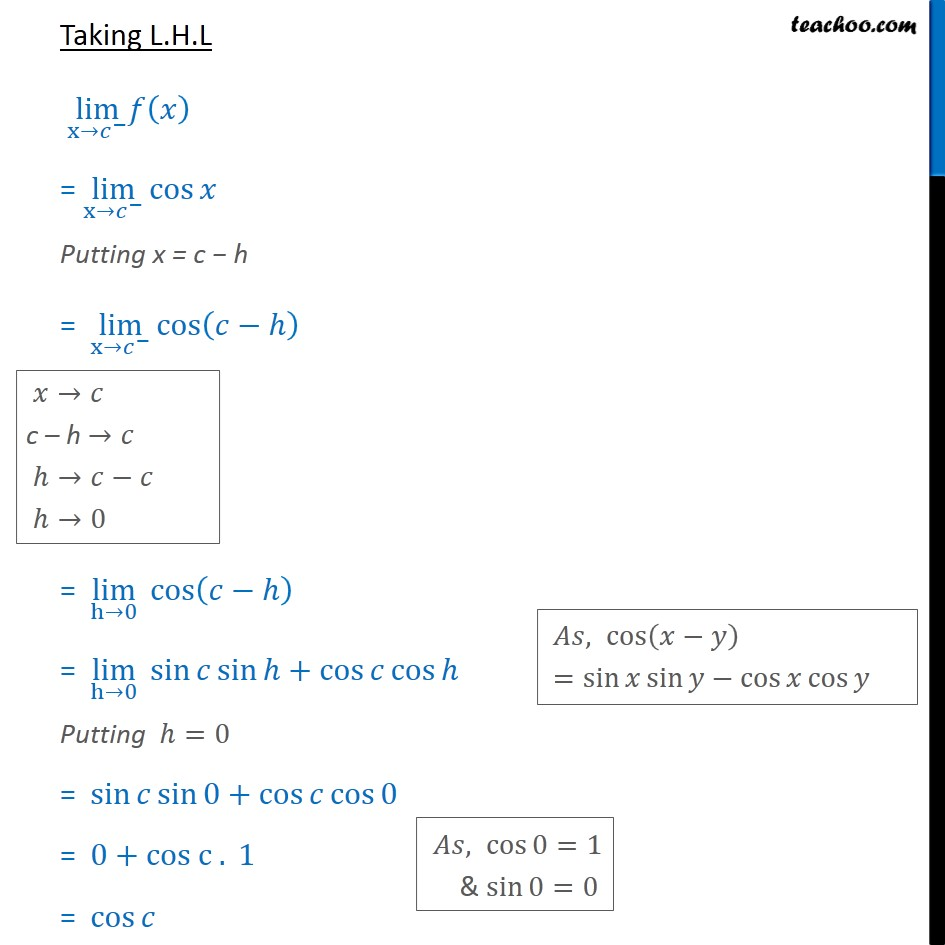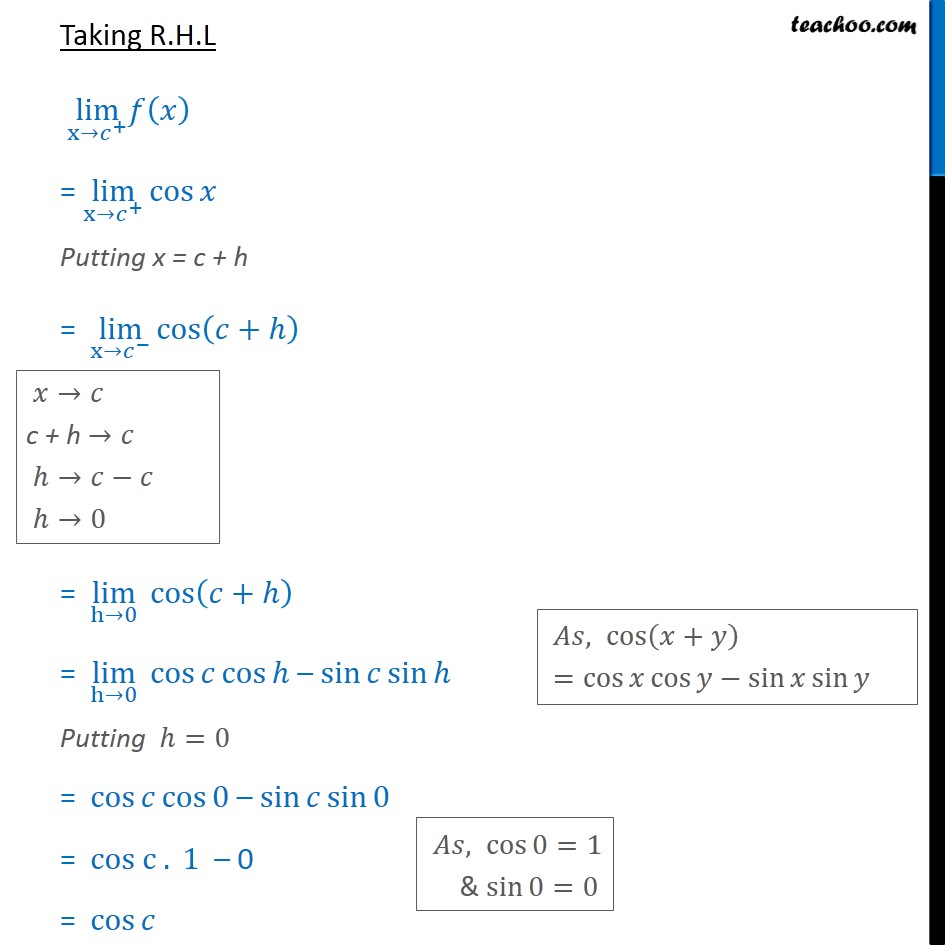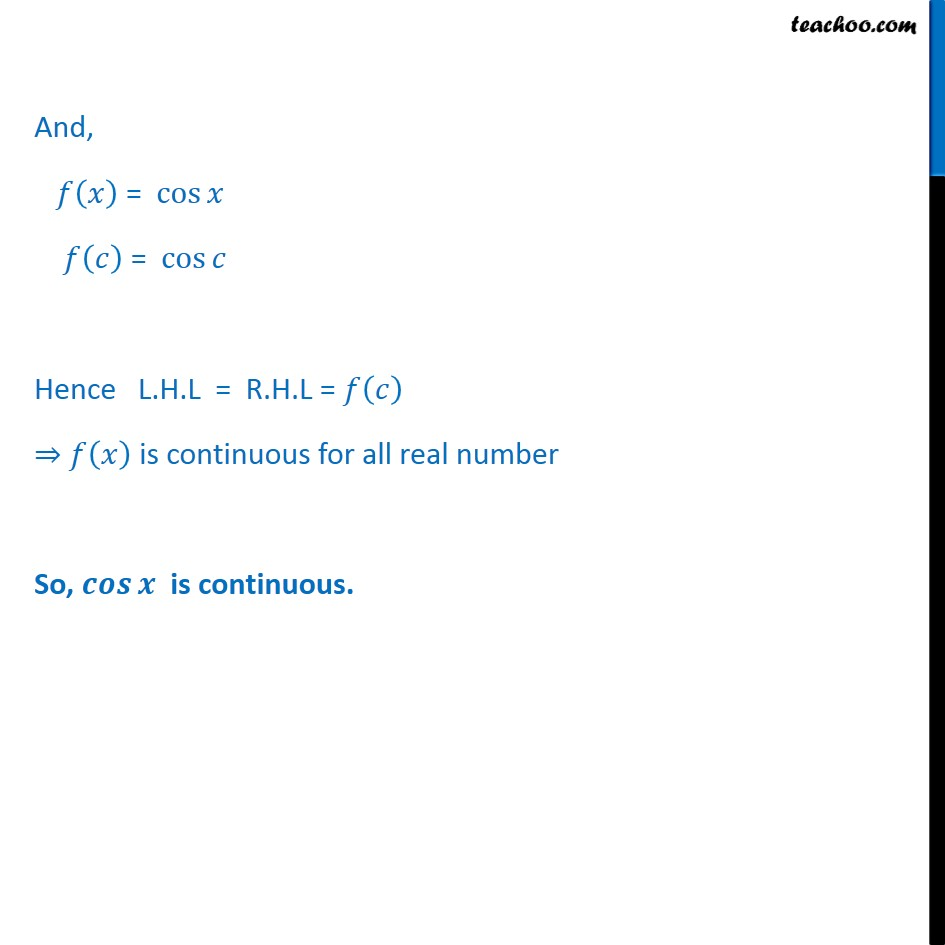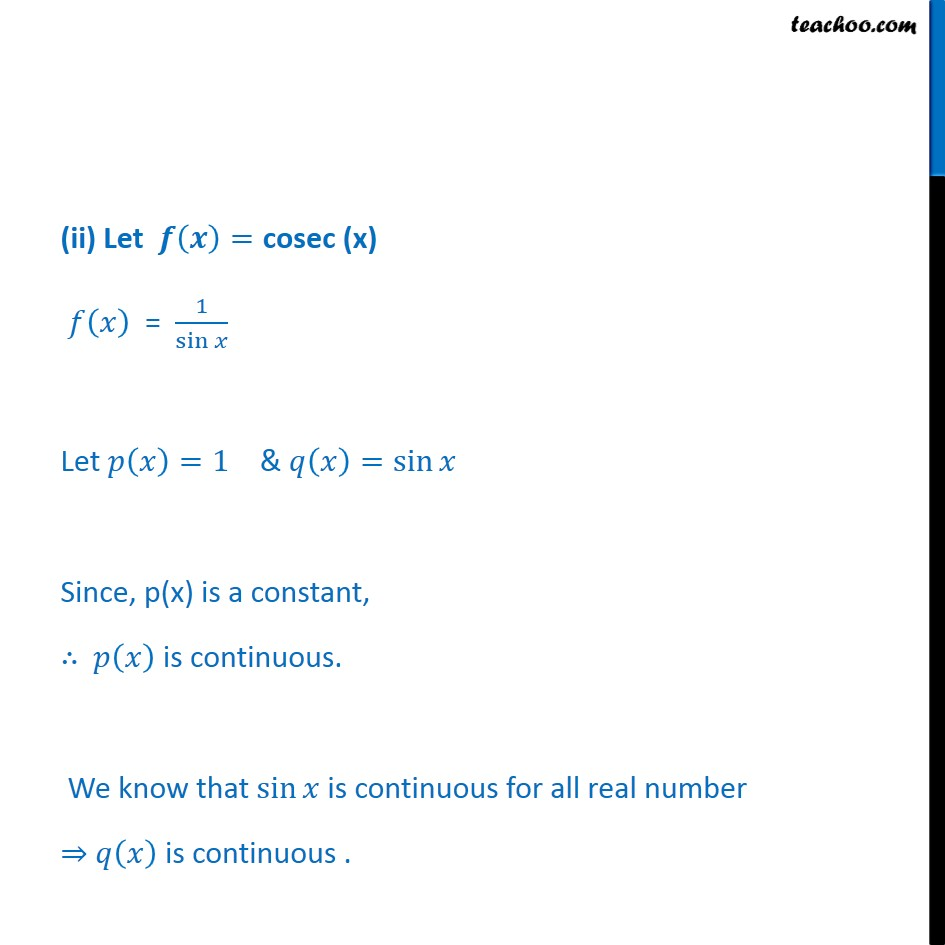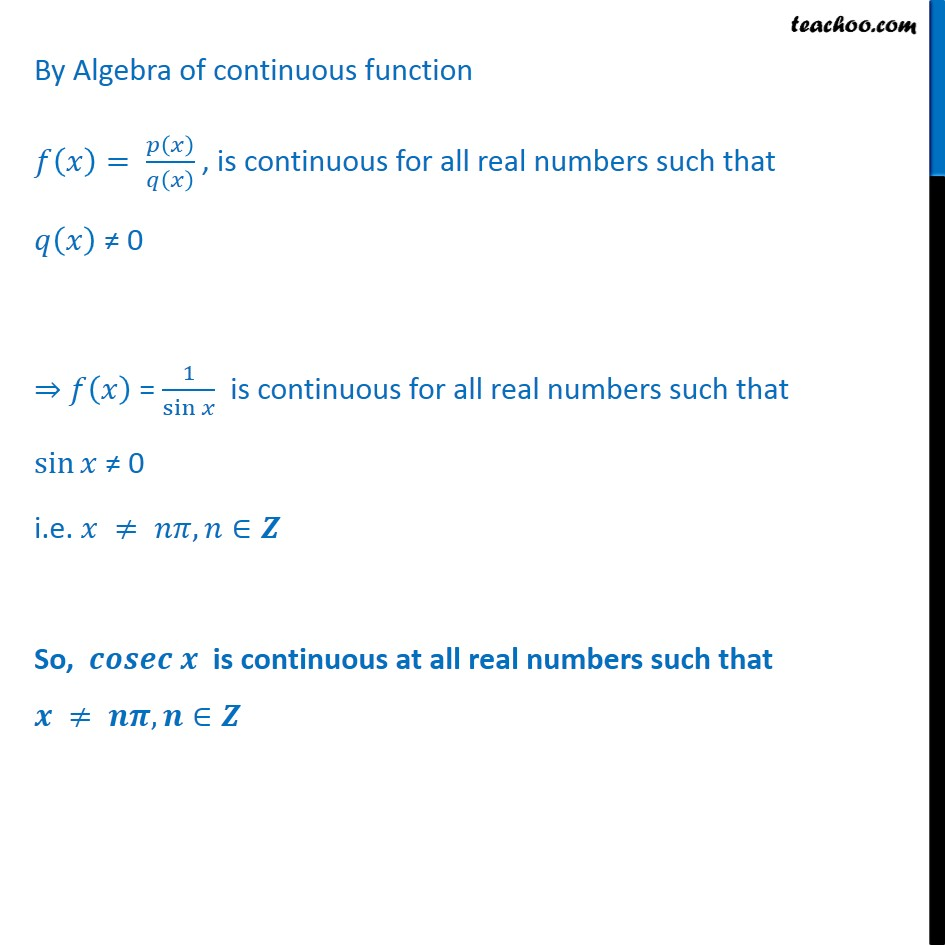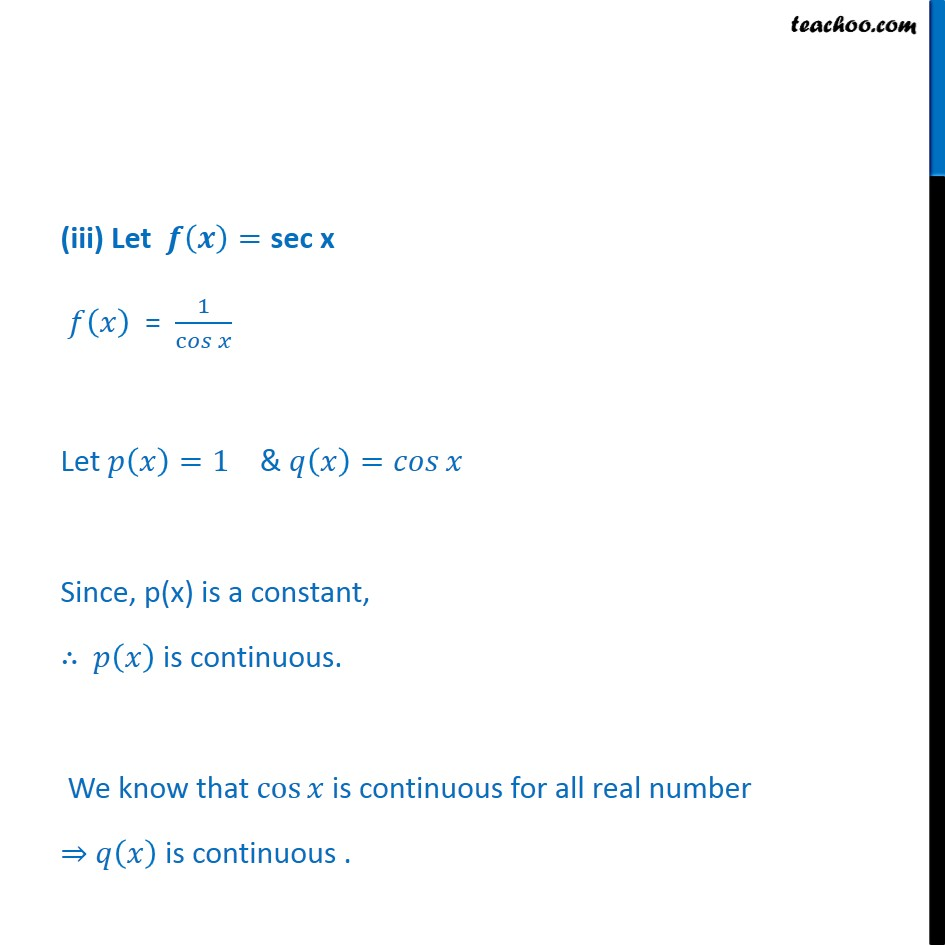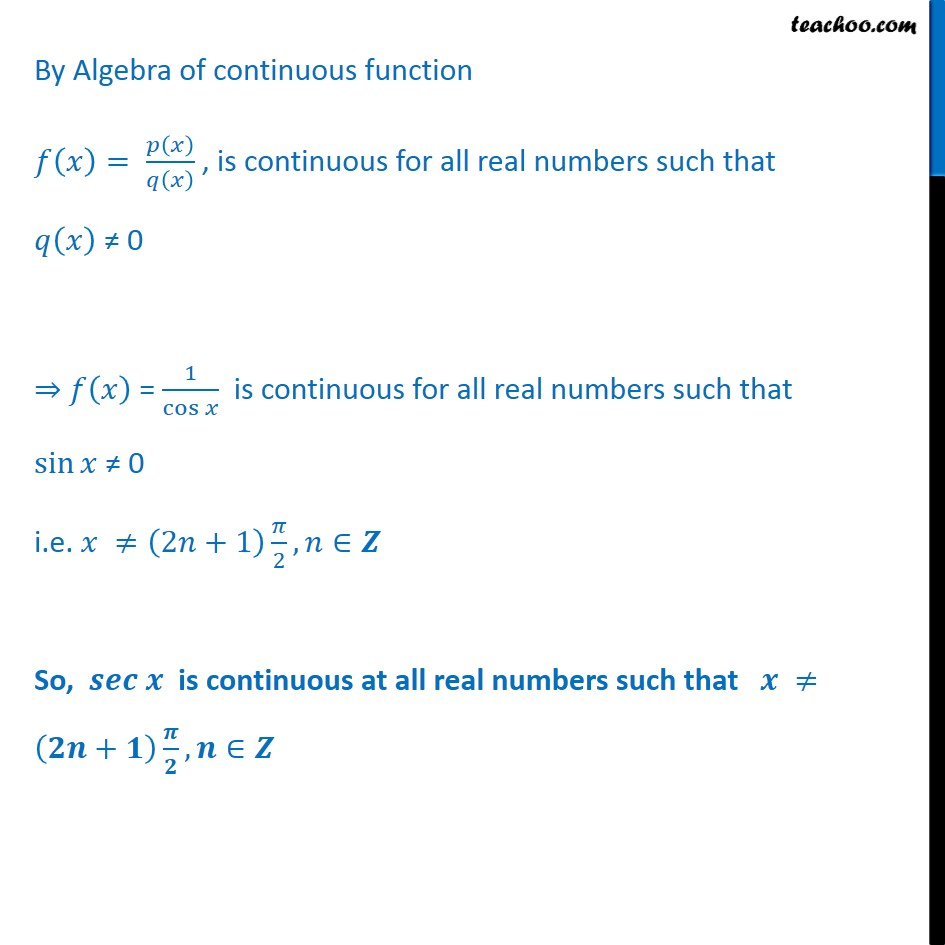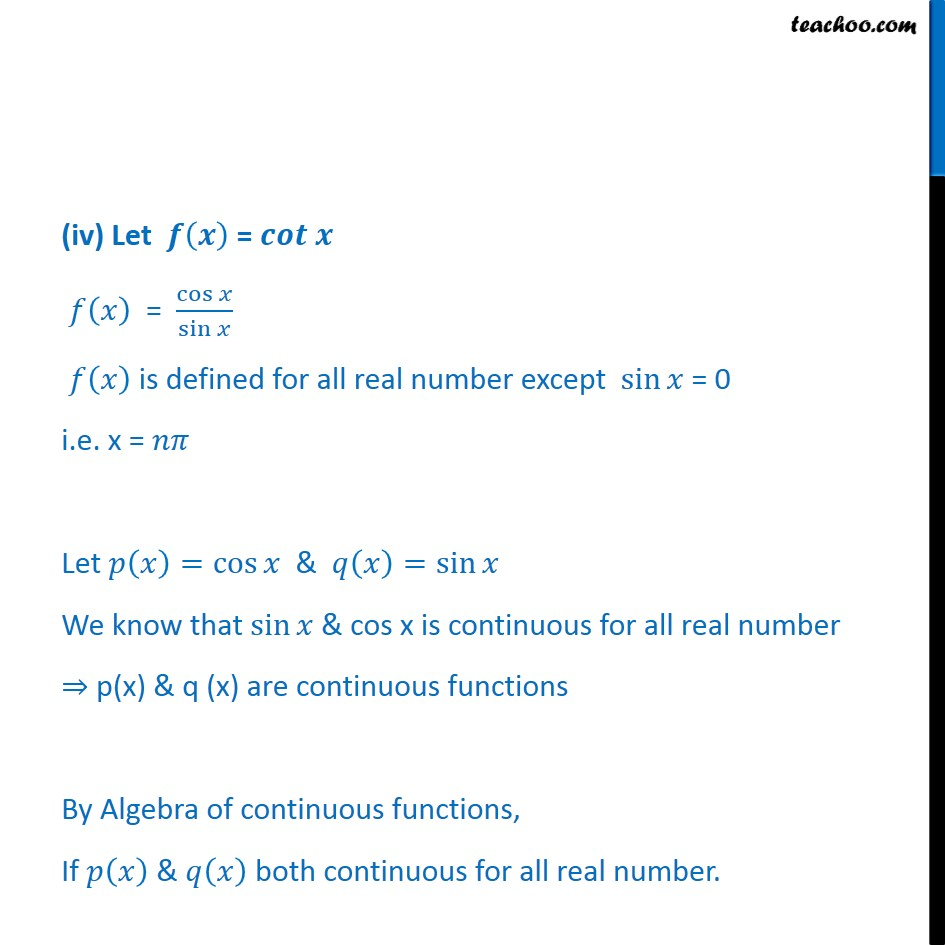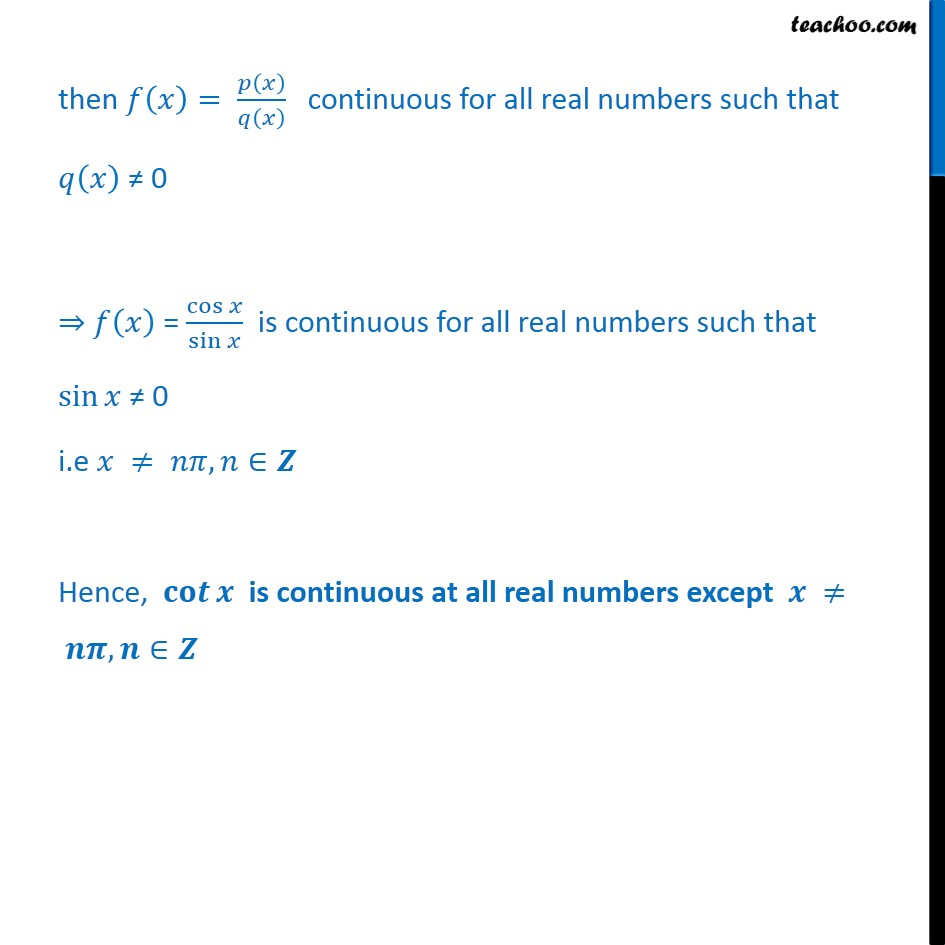1. Chapter 5 Class 12 Continuity and Differentiability
2. Serial order wise
3. Ex 5.1

Transcript

Ex 5.1, 22 Discuss the continuity of the cosine, cosecant, secant and cotangent functions. (i) Let 𝒇 𝒙﷯= 𝐜𝐨𝐬﷮𝒙﷯ Let c be any real number We know that A function is continuous at 𝑥 = 𝑐 if L.H.L = R.H.L = 𝑓 𝑐﷯ i.e. lim﷮x→ 𝑐﷮−﷯﷯ 𝑓 𝑥﷯= lim﷮x→ 𝑐﷮+﷯﷯ 𝑓 𝑥﷯= 𝑓 𝑐﷯ Taking L.H.L lim﷮x→ 𝑐﷮−﷯﷯𝑓 𝑥﷯ = lim﷮x→ 𝑐﷮−﷯﷯ cos﷮𝑥﷯ Putting x = c − h = lim﷮x→ 𝑐﷮−﷯﷯ cos﷮ 𝑐−ℎ﷯﷯ = lim﷮h→0﷯ cos﷮ 𝑐−ℎ﷯﷯ = lim﷮h→0﷯ sin﷮𝑐﷯ sin﷮ℎ﷯+ cos﷮𝑐﷯ cos﷮ℎ﷯ Putting ℎ=0 = sin﷮𝑐﷯ sin﷮0﷯+ cos﷮𝑐﷯ cos﷮0﷯ = 0+cos c . 1 = cos﷮𝑐﷯ Taking R.H.L lim﷮x→ 𝑐﷮+﷯﷯𝑓 𝑥﷯ = lim﷮x→ 𝑐﷮+﷯﷯ cos﷮𝑥﷯ Putting x = c + h = lim﷮x→ 𝑐﷮−﷯﷯ cos﷮ 𝑐+ℎ﷯﷯ = lim﷮h→0﷯ cos﷮ 𝑐+ℎ﷯﷯ = lim﷮h→0﷯ cos﷮𝑐﷯ cos﷮ℎ﷯ – sin﷮𝑐﷯ sin﷮ℎ﷯ Putting ℎ=0 = cos﷮𝑐﷯ cos﷮0﷯ – sin﷮𝑐﷯ sin﷮0﷯ = cos c . 1 – 0 = cos﷮𝑐﷯ And, 𝑓 𝑥﷯ = cos﷮𝑥﷯ 𝑓 𝑐﷯ = cos﷮𝑐﷯ Hence L.H.L = R.H.L = 𝑓 𝑐﷯ ⇒ 𝑓 𝑥﷯ is continuous for all real number So, 𝒄𝒐𝒔⁡𝒙 is continuous. (ii) Let 𝒇 𝒙﷯=cosec (x) 𝑓 𝑥﷯ = 1﷮ sin﷮𝑥﷯﷯ Let 𝑝 𝑥﷯=1 & 𝑞 𝑥﷯= sin﷮𝑥﷯ Since, p(x) is a constant, ∴ 𝑝 𝑥﷯ is continuous. We know that sin﷮𝑥﷯ is continuous for all real number ⇒ 𝑞 𝑥﷯ is continuous . By Algebra of continuous function 𝑓 𝑥﷯= 𝑝 𝑥﷯﷮𝑞 𝑥﷯﷯ , is continuous for all real numbers such that 𝑞 𝑥﷯ ≠ 0 ⇒ 𝑓 𝑥﷯ = 1﷮ sin﷮𝑥﷯﷯ is continuous for all real numbers such that sin﷮𝑥﷯ ≠ 0 i.e. 𝑥 ≠ 𝑛𝜋, 𝑛∈𝒁 So, 𝒄𝒐𝒔𝒆𝒄 𝒙 is continuous at all real numbers such that 𝒙 ≠ 𝒏𝝅, 𝒏∈𝒁 (iii) Let 𝒇 𝒙﷯=sec x 𝑓 𝑥﷯ = 1﷮ c𝑜𝑠﷮𝑥﷯﷯ Let 𝑝 𝑥﷯=1 & 𝑞 𝑥﷯= 𝑐𝑜𝑠﷮𝑥﷯ Since, p(x) is a constant, ∴ 𝑝 𝑥﷯ is continuous. We know that cos﷮𝑥﷯ is continuous for all real number ⇒ 𝑞 𝑥﷯ is continuous . By Algebra of continuous function 𝑓 𝑥﷯= 𝑝 𝑥﷯﷮𝑞 𝑥﷯﷯ , is continuous for all real numbers such that 𝑞 𝑥﷯ ≠ 0 ⇒ 𝑓 𝑥﷯ = 1﷮ cos﷮𝑥﷯﷯ is continuous for all real numbers such that sin﷮𝑥﷯ ≠ 0 i.e. 𝑥 ≠ 2𝑛+1﷯ 𝜋﷮2﷯, 𝑛∈𝒁 So, 𝒔𝒆𝒄 𝒙 is continuous at all real numbers such that 𝒙 ≠ 𝟐𝒏+𝟏﷯ 𝝅﷮𝟐﷯, 𝒏∈𝒁 (iv) Let 𝒇 𝒙﷯ = 𝒄𝒐𝒕 𝒙 𝑓 𝑥﷯ = cos﷮𝑥﷯﷮ sin﷮𝑥﷯﷯ 𝑓 𝑥﷯ is defined for all real number except sin﷮𝑥﷯ = 0 i.e. x = 𝑛𝜋 Let 𝑝 𝑥﷯= cos﷮𝑥﷯ & 𝑞 𝑥﷯= sin﷮𝑥﷯ We know that sin﷮𝑥﷯ & cos x is continuous for all real number ⇒ p(x) & q (x) are continuous functions By Algebra of continuous functions, If 𝑝 𝑥﷯ & 𝑞 𝑥﷯ both continuous for all real number. then 𝑓 𝑥﷯= 𝑝 𝑥﷯﷮𝑞 𝑥﷯﷯ continuous for all real numbers such that 𝑞 𝑥﷯ ≠ 0 ⇒ 𝑓 𝑥﷯ = cos﷮𝑥﷯﷮ sin﷮𝑥﷯﷯ is continuous for all real numbers such that sin﷮𝑥﷯ ≠ 0 i.e 𝑥 ≠ 𝑛𝜋, 𝑛∈𝒁 Hence, 𝐜𝐨𝒕﷮𝒙﷯ is continuous at all real numbers except 𝒙 ≠ 𝒏𝝅, 𝒏∈𝒁

Ex 5.1